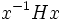# Abnormal subgroup

This article is about a definition in group theory that is standard among the group theory community (or sub-community that dabbles in such things) but is not very basic or common for people outside.
VIEW RELATED: Analogues of this | Variations of this | Opposites of this |
View a list of other standard non-basic definitions
This article defines a subgroup property: a property that can be evaluated to true/false given a group and a subgroup thereof, invariant under subgroup equivalence. View a complete list of subgroup properties[SHOW MORE]

This is an opposite of normality

## History

### Origin

This term was introduced by: Carter

The notion of abnormal subgroup was introduced by Roger W. Carter in his attempts to understand the structure of Carter subgroups of a solvable group.

## Definition

### Definition with symbols

A subgroup$H$ of a group$G$ is termed abnormal if it satisfies the following equivalent conditions:

• (Right action convention): For any$x$ in$G$,$x$ lies inside the subgroup$\langle H,H^x \rangle$. Here$H^x$ denotes the conjugate subgroup$x^{-1}Hx$.
• (Left action convention): For any$x \in G$, we have$x \in \langle H, xHx^{-1} \rangle$.
1.$H$ is a weakly abnormal subgroup of$G$ and is not contained in the intersection of two distinct conjugate subgroups.

## Relation with other properties

### Opposites

The only subgroup of a group that is both normal and abnormal is the whole group itself.

## Metaproperties

### Intermediate subgroup condition

YES: This subgroup property satisfies the intermediate subgroup condition: if a subgroup has the property in the whole group, it has the property in every intermediate subgroup.
ABOUT THIS PROPERTY: View variations of this property satisfying intermediate subgroup condition | View variations of this property not satisfying intermediate subgroup condition
ABOUT INTERMEDIATE SUBROUP CONDITION:View all properties satisfying intermediate subgroup condition | View facts about intermediate subgroup condition

If$H$ is abnormal inside$G$,$H$ is also abnormal inside$K$ for any intermediate subgroup$K$.

### Upward-closedness

This subgroup property is upward-closed: if a subgroup satisfies the property in the whole group, every intermediate subgroup also satisfies the property in the whole group
View other upward-closed subgroup properties

If$H$ is abnormal inside$G$, then so is any subgroup$K$ of$G$ containing$H$.

### Transitivity

NO: This subgroup property is not transitive: a subgroup with this property in a subgroup with this property, need not have the property in the whole group
ABOUT THIS PROPERTY: View variations of this property that are transitive|View variations of this property that are not transitive
ABOUT TRANSITIVITY: View a complete list of subgroup properties that are not transitive|View facts related to transitivity of subgroup properties | View a survey article on disproving transitivity

The property of being abnormal is not transitive. Its subordination is the property of being subabnormal.

### Trimness

The property of being abnormal is identity-true, that is, any group is abnormal as a subgroup of itself. It is not true for the trivial subgroup unless the whole group is trivial.

## Testing

### GAP code

One can write code to test this subgroup property in GAP (Groups, Algorithms and Programming), though there is no direct command for it.
View the GAP code for testing this subgroup property at: IsAbnormal
View other GAP-codable subgroup properties | View subgroup properties with in-built commands
GAP-codable subgroup property

There's no in-built function to test for abnormality, but a short snippet of code can be used to test if a subgroup is abnormal. The function is invoked as follows:

IsAbnormal(group,subgroup);